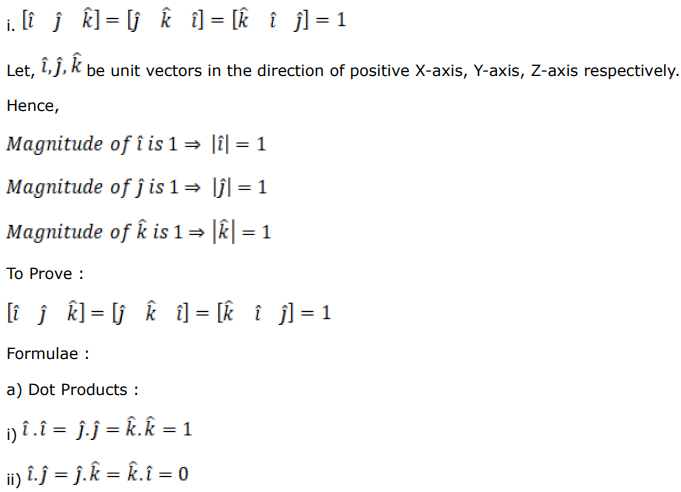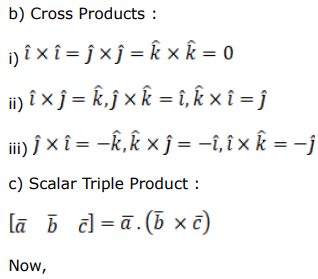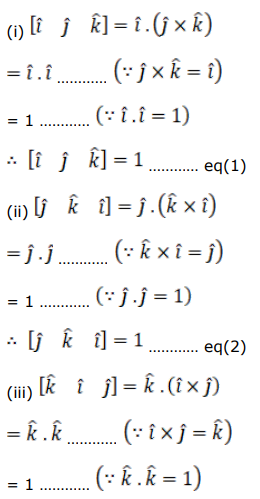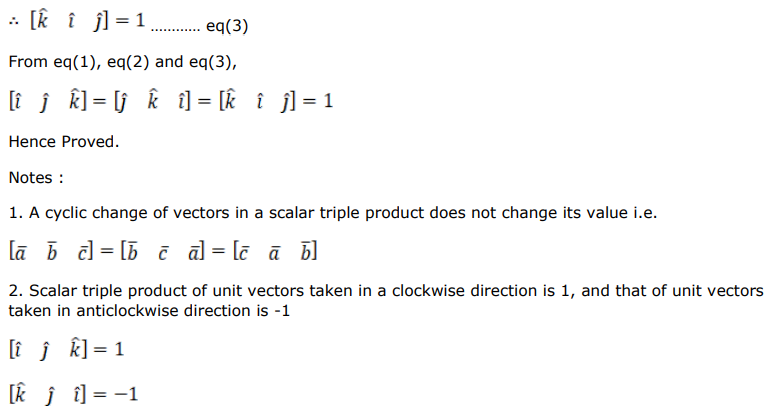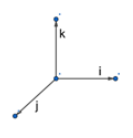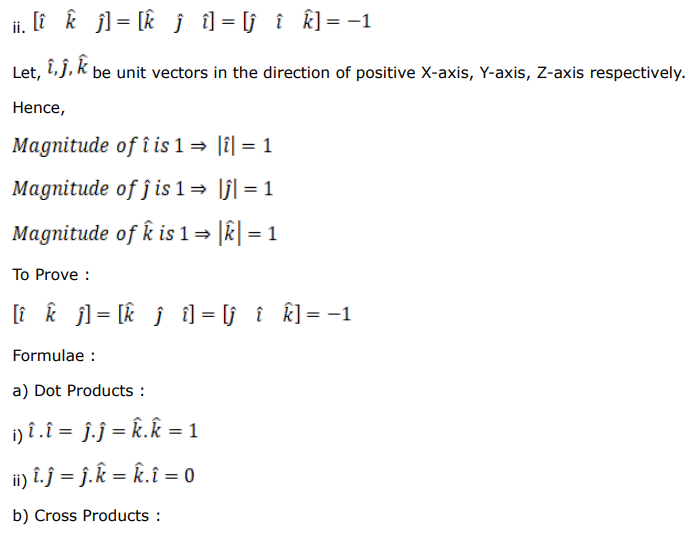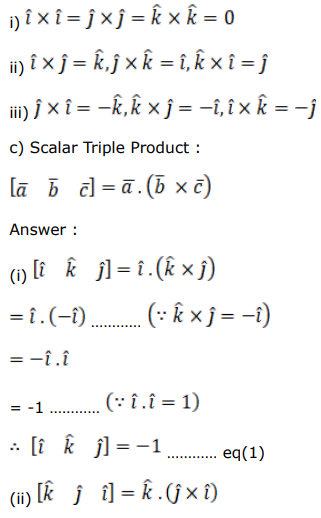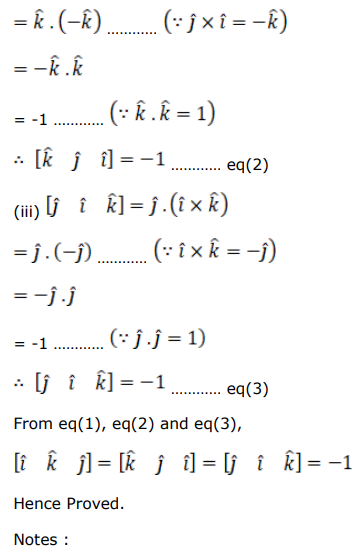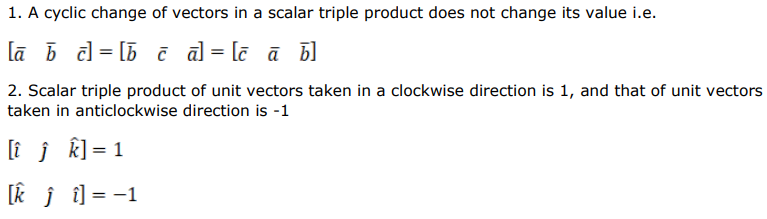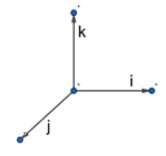# Solve this followingQuestion:

i. $\left[\begin{array}{lll}\hat{\imath} & \hat{\jmath} & \hat{k}\end{array}\right]=\left[\begin{array}{lll}\hat{\jmath} & \hat{k} & \hat{\imath}\end{array}\right]=\left[\begin{array}{lll}\hat{k} & \hat{\imath} & \hat{\jmath}\end{array}\right]=1$

ii. $\left[\begin{array}{lll}\hat{\imath} & \hat{k} & \hat{\jmath}\end{array}\right]=\left[\begin{array}{lll}\hat{k} & \hat{\jmath} & \hat{\imath}\end{array}\right]=\left[\begin{array}{lll}\hat{\jmath} & \hat{\imath} & \hat{k}\end{array}\right]=-1$

Solution: